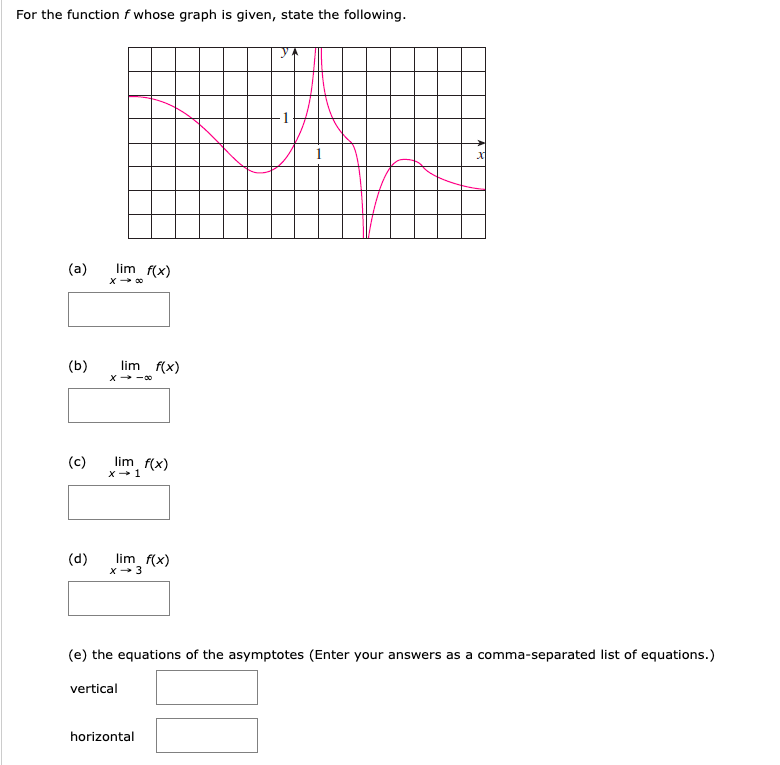# For the function f whose graph is given, state the following. lim f(x) (a) lim f(x) X o (b) lim f(x) X 1 (c) lim f(x) (d) x 3 (e) the equations of the asymptotes (Enter your answers as a comma-separated list of equations.) vertical horizontal

Questionhelp_outlineImage TranscriptioncloseFor the function f whose graph is given, state the following. lim f(x) (a) lim f(x) X o (b) lim f(x) X 1 (c) lim f(x) (d) x 3 (e) the equations of the asymptotes (Enter your answers as a comma-separated list of equations.) vertical horizontal fullscreen

1 Rating

### Want to see this answer and more?

Experts are waiting 24/7 to provide step-by-step solutions in as fast as 30 minutes!*

*Response times vary by subject and question complexity. Median response time is 34 minutes and may be longer for new subjects.
Tagged in
MathCalculus

### Limits